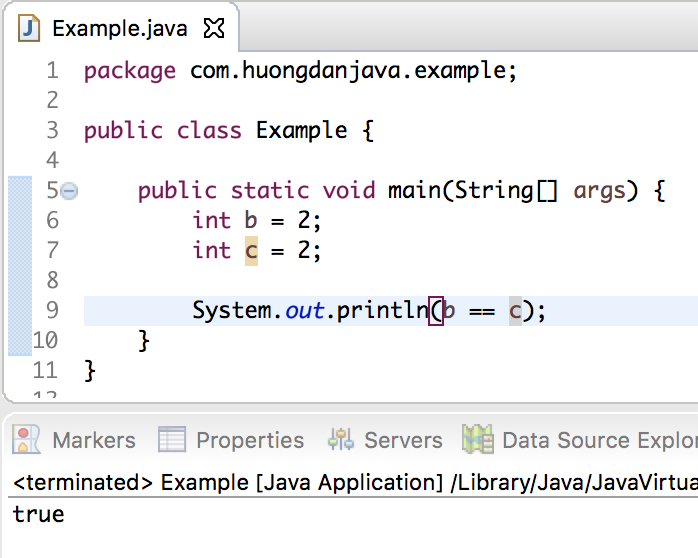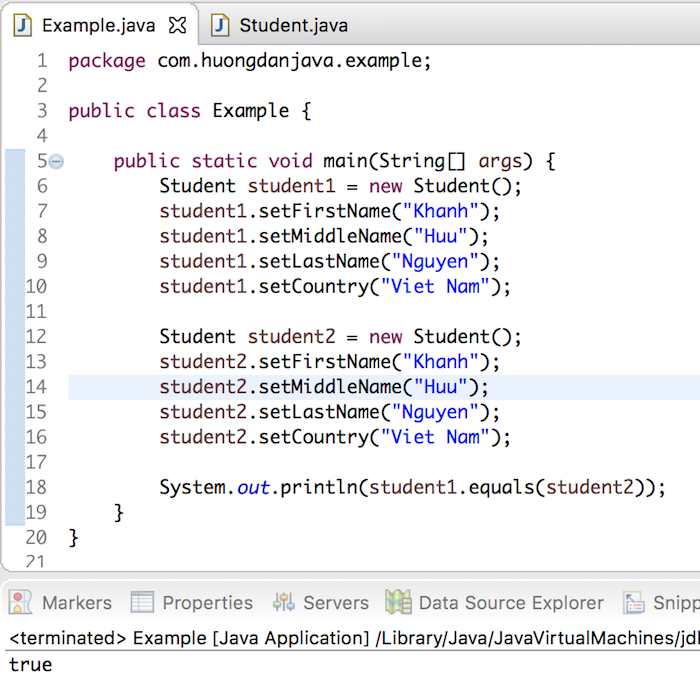# equals() and hashCode() methods in Java

The equals() and hashCode() methods are very important for all objects in Java. In this tutorial, I will present to you all the basic knowledge about these two methods.

By default, these methods are defined in Object objects, which are the parent objects of all objects in Java. But to use the right purpose of these two methods, we need to override them in our objects.

### Equals() method

This method is used to test whether two objects are equal or not?

As you know, in Java we have two basic data types, primitive and object. For primitive variables, we can use the “==” operator to compare any two variables, for example:

Result:But for the object data type, we must override the equals() method in the Object object to compare whether two objects of the same class are equal.

For example, I have a Student object like this:

Now, I will use the equals() method that the Student object inherits from Object to compare whether the two Student objects have the same firstName, middleName, lastName, and country are equal or not?

Result:As you can see, although 2 Students have the same name and country, Java does not see them as one. To solve this problem, we will override the equals() method in the Student object as follows:

In the above equals() method, I checked all the properties of the Student object. Only if all properties of two student1 and student2 objects are the same, this method returns true. And then, Java will see that these two objects are equal.

Then the result will be:### HashCode() method

Objects such as HashMap, HashTable use key objects to search for corresponding value objects. And for optimal search, Java uses hash values derived from the hashCode() method of key objects to group these objects in so-called buckets. Each bucket corresponds to a hash value.

When searching for any object in the HashMap or HashTable, Java first gets the value of the key object we want to search for. It then checks the buckets to see if the value of the hash value matches the bucket. If a bucket is satisfied, Java will return the value of the value object that we want to search.

Multiple objects can have the same hash value, and therefore they can share the same bucket. In this case, Java will use the equals() method to find exactly the value object we want to find.

Here we have a concept called contract between the equals() and hashCode() methods:

• If two objects are equal then they must have the same hashCode().
• If two objects have the same hashCode() then they can be equal or not.

5/5 - (1 vote)